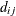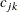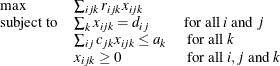### Example 6.12 An Assignment Problem

This example departs somewhat from the emphasis of previous ones. Typically, linear programming models are large, have considerable structure, and are solved with some regularity. Some form of automatic model building, or matrix generation as it is commonly called, is a useful aid. The sparse input format provides a great deal of flexibility in model specification so that, in many cases, the DATA step can be used to generate the matrix.

The following assignment problem illustrates some techniques in matrix generation. In this example, you have four machines that can produce any of six grades of cloth, and you have five customers that demand various amounts of each grade of cloth. The return from supplying a customer with a demanded grade depends on the machine on which the cloth was made. In addition, the machine capacity depends both upon the specific machine used and the grade of cloth made.

To formulate this problem, letdenote customer,denote grade, anddenote machine. Then letdenote the amount of cloth of grademade on machinefor customer; letdenote the return from selling one unit of gradecloth made on machineto customer; letdenote the demand for gradecloth by customer; letdenote the number of units of machinerequired to produce one unit of gradecloth; and letdenote the number of units of machineavailable. Then, you getThe data are saved in three data sets. The OBJECT data set contains the returns for satisfying demand, the DEMAND data set contains the amounts demanded, and the RESOURCE data set contains the conversion factors for each grade and the total amounts of machine resources available.

title 'An Assignment Problem';

data object;
input machine customer
grade1 grade2 grade3 grade4 grade5 grade6;
datalines;
1 1 102 140 105  105  125  148
1 2 115 133 118  118  143  166
1 3  70 108  83   83   88   86
1 4  79 117  87   87  107  105
1 5  77 115  90   90  105  148
2 1 123 150 125  124  154   .
2 2 130 157 132  131  166   .
2 3 103 130 115  114  129   .
2 4 101 128 108  107  137   .
2 5 118 145 130  129  154   .
3 1  83  .   .    97  122  147
3 2 119  .   .   133  163  180
3 3  67  .   .    91  101  101
3 4  85  .   .   104  129  129
3 5  90  .   .   114  134  179
4 1 108 121  79   .   112  132
4 2 121 132  92   .   130  150
4 3  78  91  59   .    77   72
4 4 100 113  76   .   109  104
4 5  96 109  77   .   105  145
;

data demand;
input customer
grade1 grade2 grade3 grade4 grade5 grade6;
datalines;
1 100 100 150  150  175  250
2 300 125 300  275  310  325
3 400   0 400  500  340    0
4 250   0 750  750    0    0
5   0 600 300    0  210  360
;

data resource;
input machine
grade1 grade2 grade3 grade4 grade5 grade6 avail;
datalines;
1 .250 .275 .300  .350  .310  .295  744
2 .300 .300 .305  .315  .320  .     244
3 .350 .    .     .320  .315  .300  790
4 .280 .275 .260  .     .250  .295  672
;


The linear program is built using the DATA step. The model is saved in a SAS data set in the sparse input format for PROC LP. Each section of the following DATA step generates a piece of the linear program. The first section generates the objective function; the next section generates the demand constraints; and the last section generates the machine resource availability constraints.

/*  build the linear programming model */

data model;
array grade{6} grade1-grade6;
length _type_ $8 _row_$ 8 _col_ \$ 8;
keep _type_ _row_ _col_ _coef_;

ncust=5;
nmach=4;
ngrade=6;

/* generate the objective function */

_type_='MAX';
_row_='OBJ';
do k=1 to nmach;
do i=1 to ncust;
link readobj;     /* read the objective coefficient data */
do j=1 to ngrade;
if grade{j}^=. then do;
_col_='X'||put(i,1.)||put(j,1.)||put(k,1.);
_coef_=grade{j};
output;
end;
end;
end;
end;

/* generate the demand constraints */

do i=1 to ncust;
link readdmd;        /* read the demand data */
do j=1 to ngrade;
if grade{j}^=. then do;
_type_='EQ';
_row_='DEMAND'||put(i,1.)||put(j,1.);
_col_='_RHS_';
_coef_=grade{j};
output;
_type_=' ';
do k=1 to nmach;
_col_='X'||put(i,1.)||put(j,1.)||put(k,1.);
_coef_=1.0;
output;
end;
end;
end;
end;

/* generate the machine constraints */

do k=1 to nmach;
link readres;       /* read the machine data */
_type_='LE';
_row_='MACHINE'||put(k,1.);
_col_='_RHS_';
_coef_=avail;
output;
_type_=' ';
do i=1 to ncust;
do j=1 to ngrade;
if grade{j}^=. then do;
_col_='X'||put(i,1.)||put(j,1.)||put(k,1.);
_coef_=grade{j};
output;
end;
end;
end;
end;

readobj: set object;
return;
readdmd: set demand;
return;
readres: set resource;
return;
run;


With the model built and saved in a data set, it is ready for solution using PROC LP. The following program solves the model and saves the solution in the data set called PRIMAL:

/* solve the linear program */

proc lp data=model sparsedata noprint primalout=primal;
run;


The following output is produced by PROC LP.

Output 6.12.1: An Assignment Problem

 An Assignment Problem

The LP Procedure

Problem Summary
Objective Function Max OBJ
Rhs Variable _RHS_
Type Variable _type_
Problem Density (%) 5.31

Variables Number

Non-negative 120
Slack 4

Total 124

Constraints Number

LE 4
EQ 30
Objective 1

Total 35

Solution Summary

Terminated Successfully
Objective Value 871426.03763

Phase 1 Iterations 0
Phase 2 Iterations 40
Phase 3 Iterations 0
Integer Iterations 0
Integer Solutions 0
Initial Basic Feasible Variables 36
Time Used (seconds) 0
Number of Inversions 3

Epsilon 1E-8
Infinity 1.797693E308
Maximum Phase 1 Iterations 100
Maximum Phase 2 Iterations 100
Maximum Phase 3 Iterations 99999999
Maximum Integer Iterations 100
Time Limit (seconds) 120

The solution is prepared for reporting using the DATA step, and a report is written using PROC TABULATE.

   /* report the solution */

data solution;
set primal;
keep customer grade machine amount;
if substr(_var_,1,1)='X' then do;
if _value_^=0 then do;
customer = substr(_var_,2,1);
grade    = substr(_var_,3,1);
machine  = substr(_var_,4,1);
amount   = _value_;
output;
end;
end;
run;

proc tabulate data=solution;
class customer grade machine;
var   amount;
table (machine*customer), (grade*amount);
run;


The report shown in Output 6.12.2 gives the assignment of customer, grade of cloth, and machine that maximizes the return and does not violate the machine resource availability.

Output 6.12.2: An Assignment Problem

 An Assignment Problem

grade
1 2 3 4 5 6
amount amount amount amount amount amount
Sum Sum Sum Sum Sum Sum
machine customer . 100.00 150.00 150.00 175.00 250.00
1 1
2 . . 300.00 . . .
3 . . 256.72 210.31 . .
4 . . 750.00 . . .
5 . 92.27 . . . .
2 3 . . 143.28 . 340.00 .
5 . . 300.00 . . .
3 2 . . . 275.00 310.00 325.00
3 . . . 289.69 . .
4 . . . 750.00 . .
5 . . . . 210.00 360.00
4 1 100.00 . . . . .
2 300.00 125.00 . . . .
3 400.00 . . . . .
4 250.00 . . . . .
5 . 507.73 . . . .